0

# 深度学习修炼（五）——基于pytorch神经网络模型进行气温预测

## 5 基于pytorch神经网络模型进行气温预测

temps.zip - 气温预测数据集下载

## 5.1 实现前的知识补充

多层感知机


### 5.1.1 神经网络的表示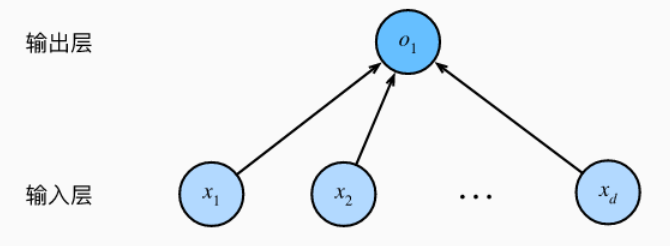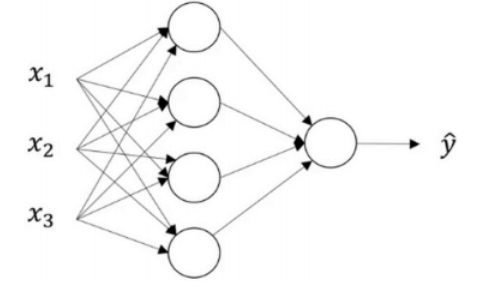x

1

,

x

2

.

.

.

x

n

x_1,x_2...x_n

x1​,x2​...xn​，它们被竖直地堆叠起来，这叫做神经网络的

输入层


。但是前面我们也说过，这一层只提供输入特征，计算不在这一层，所以一般输入层是不算入神经网络的层数的。然后这里还有另外一层我们称之为

隐藏层


；而在最后一层是输出层，他负责产生预测值。

### 5.1.4 在网络中加入隐藏层

多层感知机(multilayer perceptron)


，通常缩写为

MLP


。 下面，我们以图的方式描述了多层感知机。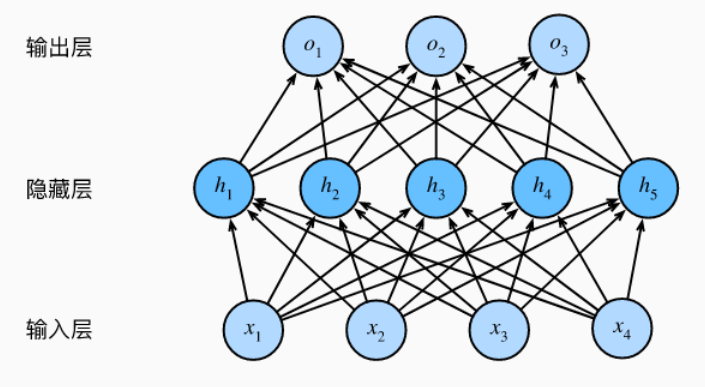### 5.1.6 小批量随机梯度下降

梯度下降(gradient descent)


小批量随机梯度下降


。如下面所示：

    (

w

,

b

)

<

−

(

w

,

b

)

−

η

∣

B

∣

∑

i

∈

B

∂

(

w

,

b

)

l

(

i

)

(

w

,

b

)

(w,b)<-(w,b)-\frac{η}{|B|} \sum _{i∈B}∂_{(w,b)}l^{(i)}(w,b)

(w,b)<−(w,b)−∣B∣η​∑i∈B​∂(w,b)​l(i)(w,b)


    B

B

B个样本，也就是小批量

B

B

B。参数η我们叫做学习率。


## 5.2 实现的过程

### 5.2.1 预处理

import pandas as pd
import numpy as np
import torch
import matplotlib.pyplot as plt


features = pd.read_csv('temps.csv')# 查看数据前五行


out：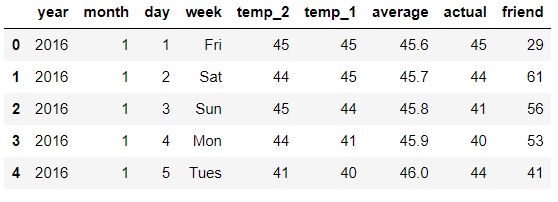• temp_2：前天的最高温度值
• temp_1：昨天的最高温度值
• average：在历史中，每年这一天的平均最高温度值
• actual：标签值，当天的真实最高温度
• friend：朋友预测的可能值

# 查看数据的维度print('数据维度：',features.shape)


out：

# 将时间转换为时间序列import datetime

# 分别得到年、月、日
years = features['year']
months = features['month']
days = features['day']# datetime格式
dates =[str(int(year))+'-'+str(int(month))+'-'+str(int(day))for year,month,day inzip(years,months,days)]
dates =[datetime.datetime.strptime(date,'%Y-%m-%d')for date in dates]


dates[:5]


out：

[datetime.datetime(2016, 1, 1, 0, 0),
datetime.datetime(2016, 1, 2, 0, 0),
datetime.datetime(2016, 1, 3, 0, 0),
datetime.datetime(2016, 1, 4, 0, 0),
datetime.datetime(2016, 1, 5, 0, 0)]

# 将week转为独热编码
features = pd.get_dummies(features)


out：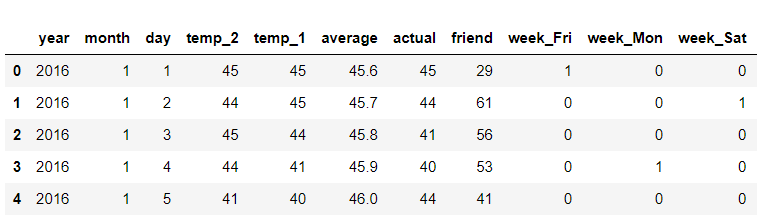# 分离y_train
labels = np.array(features['actual'])# 在特征中去掉y_train
features = features.drop('actual',axis =1)# 名字单独保存一下，以备后患
feature_list =list(features.columns)# 转换成合适的格式
features = np.array(features)


features.shape


out：

(348, 14)

#有些数值大有些数值小，做标准化from sklearn import preprocessing
input_features = preprocessing.StandardScaler().fit_transform(features)


### 5.2.2 搭建网络模型

# 将array转为张量
x = torch.tensor(input_features,dtype =float)
y = torch.tensor(labels,dtype =float)


# 权重参数初始化


learning_rate =0.001
losses =[]


# 指定梯度下降次数1000次for i inrange(1000):# 计算隐藏层-线性模型
hidden = x.mm(weights)+ biases
# 加入激活函数-加入激活函数
hidden = torch.relu(hidden)# 预测结果计算-输出值
predictions = hidden.mm(weights2)+ biases2
# 计算损失-平方损失函数
loss = torch.mean((predictions - y)**2)# 将每次的损失转为numpy格式并添加到损失列表中
losses.append(loss.data.numpy())# 打印损失值if i %100==0:print('loss:',loss)# 反向传播计算
loss.backward()# 更新参数


out：

## 5.3 简化实现

# 指定规模
input_size = input_features.shape
hidden_size =128
output_size =1
batch_size =16# 搭建网络
my_nn = torch.nn.Sequential(
torch.nn.Linear(input_size,hidden_size),
torch.nn.Sigmoid(),
torch.nn.Linear(hidden_size,output_size),)# 定义损失函数
cost = torch.nn.MSELoss(reduction ='mean')

# 训练网络
losses =[]for i inrange(1000):
batch_loss =[]# 小批量随机梯度下降进行训练for start inrange(0,len(input_features),batch_size):
end = start+batch_size if start + batch_size <len(input_features)elselen(input_features)
xx = torch.tensor(input_features[start:end],dtype = torch.float,requires_grad =True)
yy = torch.tensor(labels[start:end],dtype = torch.float,requires_grad =True)
prediction = my_nn(xx)
loss = cost(prediction,yy)
loss.backward(retain_graph=True)
optimizer.step()
batch_loss.append(loss.data.numpy())# 打印损失# 打印损失值if i %100==0:
losses.append(np.mean(batch_loss))print(i,np.mean(batch_loss))


out:

0 3984.4104
100 37.5414
200 35.608612
300 35.26231
400 35.09969
500 34.969772
600 34.853214
700 34.73715
800 34.61541
900 34.48683

## 5.4 评估模型

x = torch.tensor(input_features,dtype = torch.float)
predict = my_nn(x).data.numpy()# 转换日期格式
dates =[str(int(year))+'-'+str(int(month))+'-'+str(int(day))for year,month,day inzip(years,months,days)]
dates =[datetime.datetime.strptime(date,'%Y-%m-%d')for date in dates]# 创建一个表格来存日期和其对应的标签数值
true_data = pd.DataFrame(data ={'date':dates,'actual':labels})# 同理，在创建一个来存日期和其对应的模型预测值
mouths = features[:,feature_list.index('month')]
days = features[:,feature_list.index('day')]
years = features[:,feature_list.index('year')]

test_dates =[str(int(year))+'-'+str(int(month))+'-'+str(int(day))for year,month,day inzip(years,months,days)]
test_dates =[datetime.datetime.strptime(date,'%Y-%m-%d')for date in test_dates]

predictions_data = pd.DataFrame(data ={'date':test_dates,'prediction':predict.reshape(-1)})# 真实值
plt.plot(true_data['date'],true_data['actual'],'b-',label ='actual')# 预测值
plt.plot(predictions_data['date'],predictions_data['prediction'],'ro',label ='prediction')
plt.xticks(rotation ='60')
plt.legend()# 图名
plt.xlabel('Date');plt.ylabel('Maximum Temperature (F)');plt.title('Actual and Predicted Values')

plt.show()


out：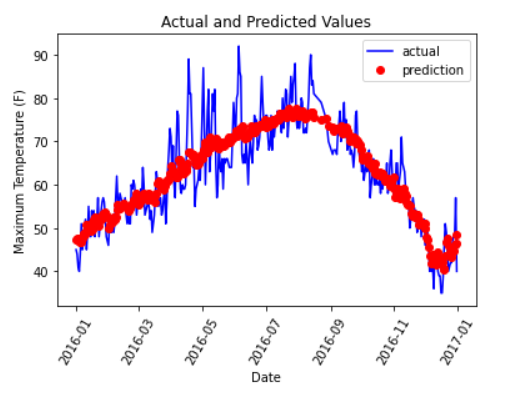### “深度学习修炼（五）——基于pytorch神经网络模型进行气温预测”的评论:

##### 关于作者##### overfit同步小助手